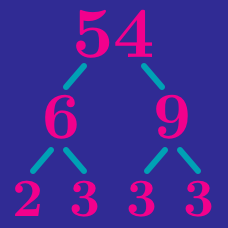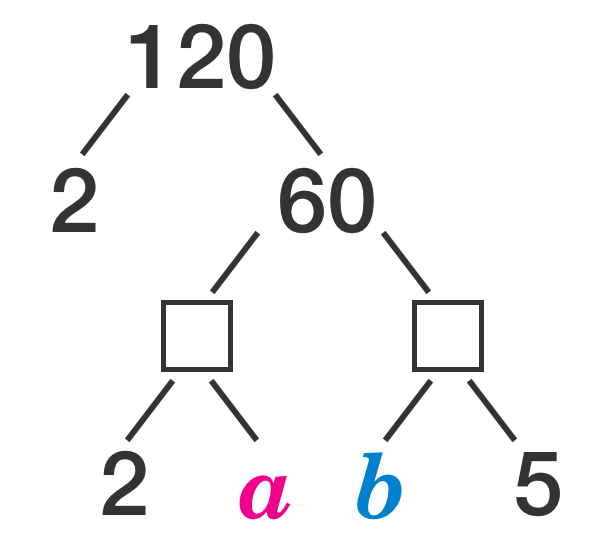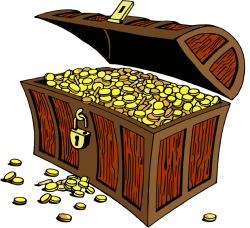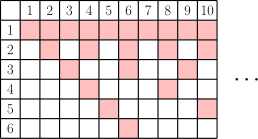Number Theory

# Prime Factorization Warmup

What is the smallest positive integer divisible by each of 10, 20, and 30?

What is $a \times b \, ?$A group of explorers discovers a chest containing 5040 gold coins. When they try to divide the coins so that each explorer receives the same number of coins, they find that they are unable to do so. Which of these could be the number of explorers in the group?

Hint: $5040 = 7! = 1 \times 2 \times 3 \times 4 \times 5 \times 6 \times 7.$

What is the smallest number that is divisible by 1, 2, 3, 4, 5 and 6?Which of these statements are true?

I. Even numbers are never divisible by odd numbers.

II. Odd numbers are never divisible by even numbers.

×